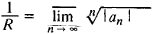# Circle of Convergence

## circle of convergence

[′sər·kəl əv kən′vər·jəns]
(mathematics)
The region in which a power series possesses a limit.

## Circle of Convergence

The circle of convergence of a power series

(*) a0 + a1(z - z0)2 + a2(z - z0)2 + …

is a circle ǀz – z0ǀ = R in the plane of the complex variable z such that the series (*) con verges if ǀz – aǀ < R and diverges if ǀz – aǀ > R (the series may both converge and diverge at points on the circle ǀz – z0ǀ = R). Every power series converges in the entire plane (for any z), has a circle of convergence of finite radius R, or converges only when z = Z0. The series (*) converges to an analytic function in the circle of convergence. The number R is called the radius of convergence of the series (*) and determined from the Cauchy-Hadamard formulaIf z0 = x0 is a real number, then the part of the real axis Ox lying in the circle of convergence is called the interval of convergence.

Site: Follow: Share:
Open / Close